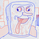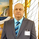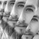962 görüntülenme
962
This is an experimental study which calculates a linear regression channel over a specified period or interval using custom moving average types for its calculations.

Linear regression is a linear approach to modeling the relationship between a dependent variable and one or more independent variables.
In linear regression , the relationships are modeled using linear predictor functions whose unknown model parameters are estimated from the data.

The regression channel in this study is modeled using the least squares approach with four base average types to choose from:
-> Arnaud Legoux Moving Average ( ALMA )
-> Exponential Moving Average ( EMA )
-> Simple Moving Average ( SMA )
-> Volume Weighted Moving Average ( VWMA )
When using VWMA , if no volume is present, the calculation will automatically switch to tick volume , making it compatible with any cryptocurrency, stock, currency pair, or index you want to analyze.

There are two window types for calculation in this script as well:
-> Continuous, which generates a regression model over a fixed number of bars continuously.
-> Interval, which generates a regression model that only moves its starting point when a new interval starts. The number of bars for calculation cumulatively increases until the end of the interval.

The channel is generated by calculating standard deviation multiplied by the channel width coefficient, adding it to and subtracting it from the regression line, then dividing it into quartiles.

To observe the path of the regression, I've included a tracer line, which follows the current point of the regression line. This is also referred to as a Least Squares Moving Average ( LSMA ).

For added predictive capability, there is an option to extend the channel lines into the future.

A custom bar color scheme based on channel direction and price proximity to the current regression value is included.

I don't necessarily recommend using this tool as a standalone, but rather as a supplement to your analysis systems.
Regression analysis is far from an exact science. However, with the right combination of tools and strategies in place, it can greatly enhance your analysis and trading.Thank you for the new script once again. I'm a big fan!
Cevap GönderWhat three parameters we are expecting here?

//Correlation Coefficient
corr(x, y, t)=>

Close, length and something else?

Thanks.
Cevap Göndersyracusepro
@syracusepro, Great question. x is the bar number counting upward, y is the price value, and t is the sampling length. The slope parameter b is dependent on correlation between price and time.
Cevap Gönder